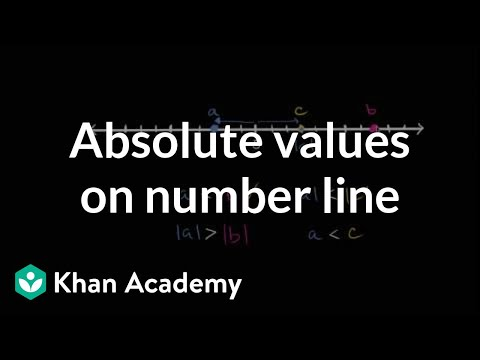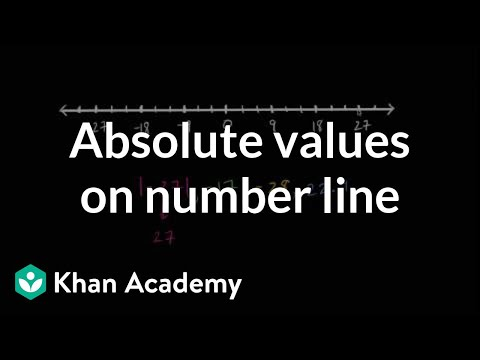Video

# Negative numbers, variables, number line (Full video)

Description: Given a, b, and c shown on the number line, Sal determines if statements like -b < c are true. So, to the left of the number line we have the number a, and then we have the number b here, a little bit closer to zero, and then on the right side of zero we have the number c. So, let's think about where negative b is on this number line. So if b is one hash mark to the left of zero, negative b is going to be one hash mark to the right of zero. C is one, two, three, four, five hash marks to the right of zero.

### Other videos you might be interested in### Comparing absolute values on the number line (Full video)